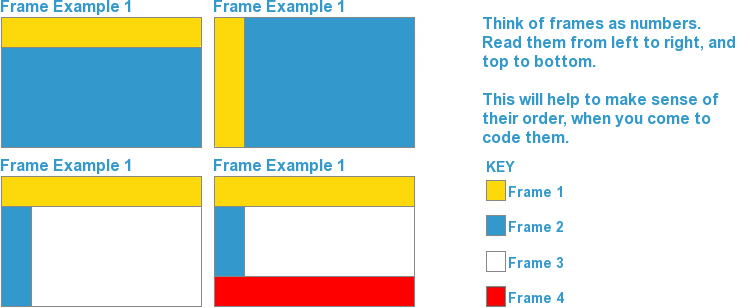Welcome to S-Design
HTML TUTORIALS

HTML Frame 2

In the previous HTML Frames chapter we demonstrated how to code Frame Example 1.
Here we'll demonstrate Frame Example 2. The code for Frame Example 3 & 4 is also below, but we'll let you figure out how & why they're coded the way they are.

To recap, here is the example of the most common framsets:Lets look at Frame Example 2 (above):

You will see that the page is made up of 2 vertical COLUMNS.
We need to tell the <frameset> this, by adding cols="64,*".
This tells the browser to split the webpage into 2 vertical columns (frames) - the first one having a width of (64), and the other taking up what space is left (*).
Example: `<frameset cols="64,*">`

From the previous HTML Frames chapter, you should already know how to specify the properties of each frame, so we'll skip the explanation.
For Frame Example 2 (above), this is what your code would look like now: ``` <html> <head> <title></title> </head> <frameset cols="64,*"> <frame name="menu" scrolling="no" noresize="noresize" src="menu.html" frameborder="0"> <frame name="content" scrolling="yes" noresize="noresize" src="welcome.html" frameborder="0"> <noframes> <body> <p>This page uses frames, but your browser doesn't support them!</p> </body> </noframes> </frameset> </html> ```

The code for Frame Example 3 (above) is as follows: ``` <html> <head> <title></title> </head> <frameset rows="64,*"> <frame name="logo" scrolling="no" noresize="noresize" src="logo.html" frameborder="0"> <frameset cols="64,*"> <frame name="menu" scrolling="no" noresize="noresize" src="menu.html" frameborder="0"> <frame name="content" scrolling="yes" noresize="noresize" src="welcome.html" frameborder="0"> </frameset> <noframes> <body> <p>This page uses frames, but your browser doesn't support them!</p> </body> </noframes> </frameset> </html> ```

The code for Frame Example 4 (above) is as follows: ``` <html> <head> <title></title> </head> <frameset rows="64,*,64"> <frame name="logo" scrolling="no" noresize="noresize" src="logo.html" frameborder="0"> <frameset cols="64,*"> <frame name="menu" scrolling="no" noresize="noresize" src="menu.html" frameborder="0"> <frame name="content" scrolling="yes" noresize="noresize" src="welcome.html" frameborder="0"> </frameset> <frame name="footer" scrolling="no" noresize="noresize" src="footer.html" frameborder="0"> <noframes> <body> <p>This page uses frames, but your browser doesn't support them!</p> </body> </noframes> </frameset> </html> ```

Now you know how to create Frames, lets have a look at Inline Frames in HTML Frames 3.

Title